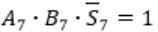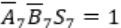October 14, 2023
October 14, 2023
October 14, 2023
###### GATE 2007
October 14, 2023
 Question 9

When two 8-bit numbers A7…A0 and B7…B0 in 2’s complement representation (with A0 and B0 as the least significant bits) are added using a ripple-carry adder, the sum bits obtained are S7…S0 and the carry bits are C7…C0. An overflow is said to have occurred if

 A the carry bit C7 is 1 B all the carry bits (C7,…,C0) are 1 CDQuestion 9 Explanation:
⇾ Overflow may occur when numbers of same sign are added
i.e., A7 = B7
⇾ Overflow can be detected by checking carry into the sign bits (Cin) and carry out of the sign bits (Cout).
⇾ Overflow occurs iff A7 = B7 and Cin ≠ Cout
These conditions are equivalent toConsiderHere A7 = B7 = 1 and S7 = 0
This happens only if Cin = 0Carry out Cout=1 whenSimilarly, in case ofCin=1 and Cout will be 0.Question 9 Explanation:
⇾ Overflow may occur when numbers of same sign are added
i.e., A7 = B7
⇾ Overflow can be detected by checking carry into the sign bits (Cin) and carry out of the sign bits (Cout).
⇾ Overflow occurs iff A7 = B7 and Cin ≠ Cout
These conditions are equivalent toConsiderHere A7 = B7 = 1 and S7 = 0
This happens only if Cin = 0Carry out Cout=1 whenSimilarly, in case ofCin=1 and Cout will be 0.